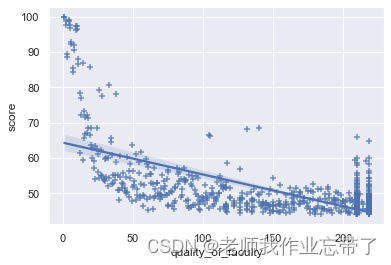# 回归分析预测世界大学综合得分

## 世界大学综合得分预测

### 数据观察与处理：

``````import pandas as pd
import numpy as np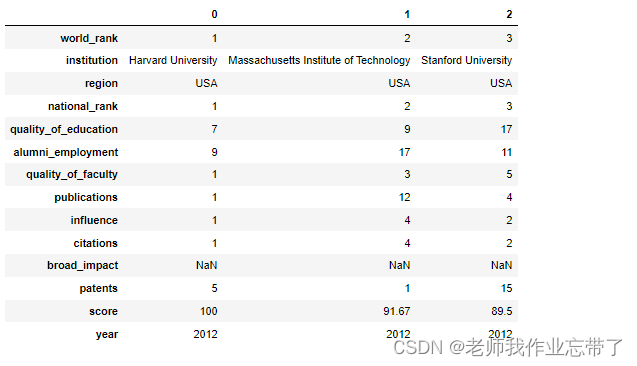``````data_df = data_df.dropna()
len(data_df) # 2000``````

### 设置矩阵

``````feature_cols = ['quality_of_faculty', 'publications', 'citations', 'alumni_employment',
'influence', 'quality_of_education', 'broad_impact', 'patents'] # 提取特征值
X = data_df[feature_cols]
Y = data_df['score']
# X Y分别为自变量 因变量矩阵``````

### 数据可视化

``````import matplotlib.pyplot as plt
import seaborn as sns

mean_df = data_df.groupby('institution').mean()  # 按学校聚合并对聚合的列取平均
top_df = mean_df.sort_values(by='score', ascending=False).head(10)  # 取前十学校
sns.set()
x = top_df['score'].values  # 综合得分列表
y = top_df.index.values  # 学校名称列表
sns.barplot(x, y, orient='h', palette="Blues_d")  # 画条形图
plt.xlim(75, 101)  # 限制 x 轴范围
plt.show()```````pairplot`的方法观察变量之间的关联关系，可以从图中看到，少部分变量之间有线性关系；各个变量和结果之间，近似对数关系。

``````sns.pairplot(data_df[feature_cols + ['score']], height=3, diag_kind="kde")
plt.show()``````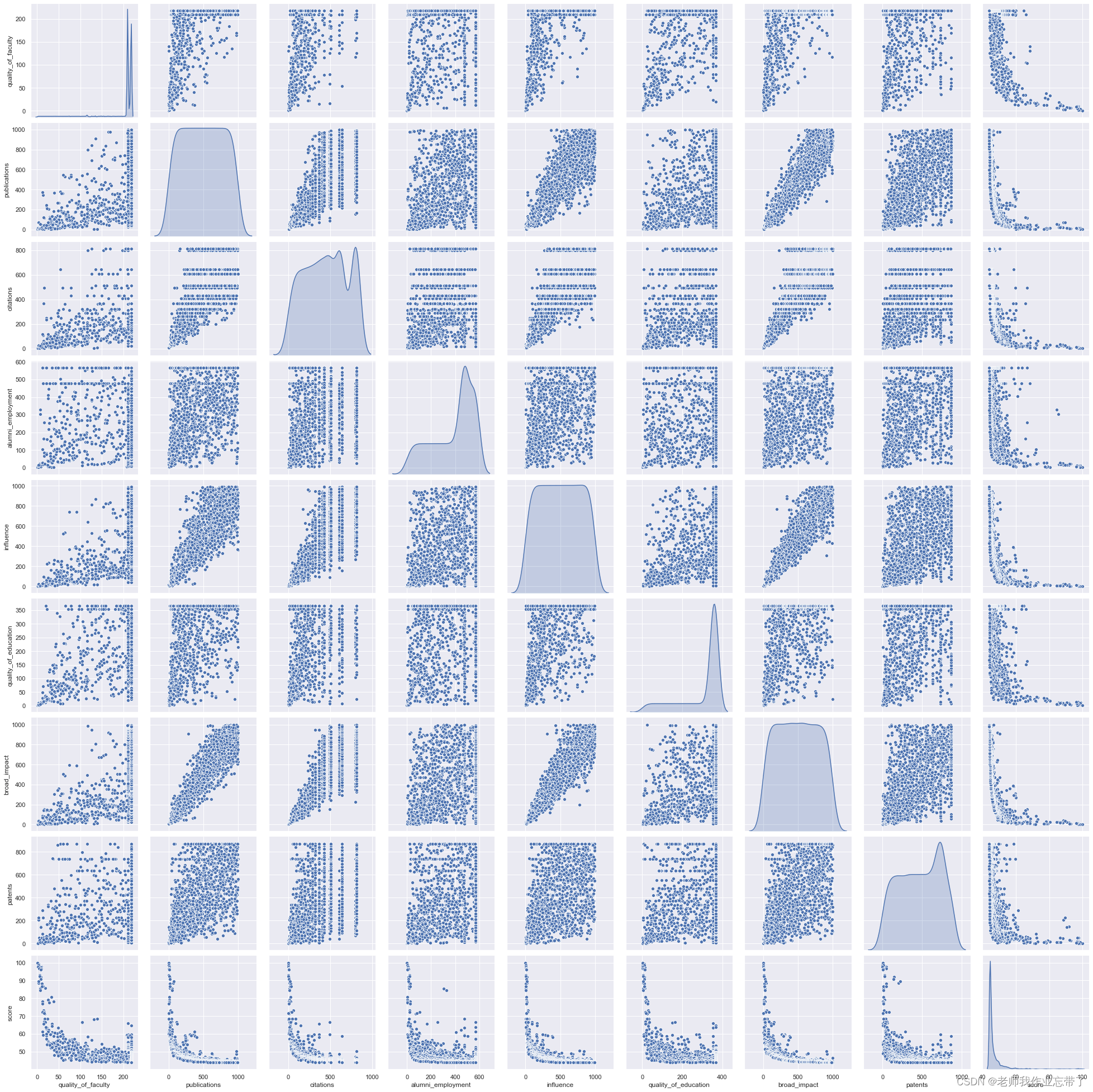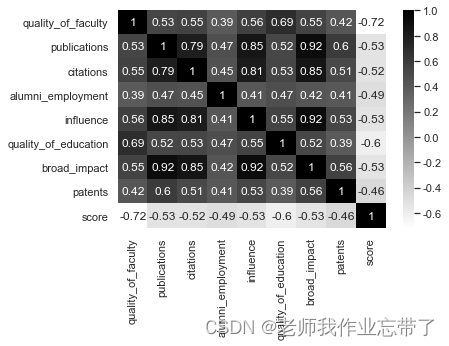### 构建模型

``````all_y = data_df['score'].values
all_x = data_df[feature_cols].values
# 取 values 是为了从 pandas 的 Series 转成 numpy 的 array

from sklearn.model_selection import train_test_split
x_train, x_test, y_train, y_test = train_test_split(all_x, all_y, test_size=0.2, random_state=2020)
all_y.shape, all_x.shape, x_train.shape, x_test.shape, y_train.shape, y_test.shape # 输出数据行列信息
# ((2000,), (2000, 8), (1600, 8), (400, 8), (1600,), (400,))``````
``````from sklearn.linear_model import LinearRegression
LR = LinearRegression()  # 线性回归模型
LR.fit(x_train, y_train)  # 在训练集上训练
p_test = LR.predict(x_test)  # 在测试集上预测，获得预测值
test_error = p_test - y_test  # 预测误差
test_rmse = (test_error**2).mean()**0.5  # 计算 RMSE
'rmse: {:.4}'.format(test_rmse)

# rmse: 3.999``````

``````import matplotlib.pyplot as plt
import seaborn as sns

sns.set()
sns.barplot(x=LR.coef_, y=feature_cols)
plt.show()``````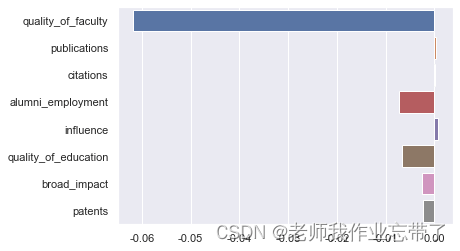``````sns.regplot(data_df['quality_of_faculty'], data_df['score'], marker="+")
plt.show()``````